# Energy, Work, and Power

## Basic Electricity

• #### Question 1

Define the following terms: energy, work, and power.

• #### Question 2

For a given amount of electric current, which resistor will dissipate the greatest amount of power: a small value (low-resistance) resistor, or a high value (high-resistance) resistor? Explain your answer.

• #### Question 3

Plot the relationship between power and current for a 2 Ω resistor on this graph: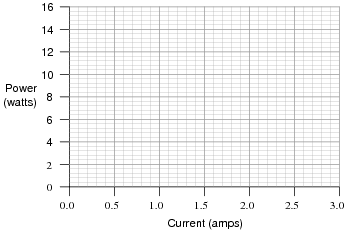What pattern do you see represented by plot? How does this compare with the graphical relationship between voltage and current for a resistor?

• #### Question 4

Shown here is a schematic diagram for a simple battery-powered flashlight:What could be modified about the circuit or its components to make the flashlight produce more light when turned on?

• #### Question 5

There are two basic Ohm’s Law equations: one relating voltage, current, and resistance; and the other relating voltage, current, and power (the latter equation is sometimes known as Joule’s Law rather than Ohm’s Law):

 E = I R

 P = I E

In electronics textbooks and reference books, you will find twelve different variations of these two equations, one solving for each variable in terms of a unique pair of two other variables. However, you need not memorize all twelve equations if you have the ability to algebraically manipulate the two simple equations shown above.

Demonstrate how algebra is used to derive the ten “other” forms of the two Ohm’s Law / Joule’s Law equations shown here.

• #### Question 6

Suppose someone mechanically couples an electric motor to an electric generator, then electrically couples the two devices together in an effort to make a perpetual-motion machine:Why won’t this assembly spin forever, once started?

• #### Question 7

Suppose a mass is connected to a winch by means of a cable, and a person turns the winch drum to raise the mass off the ground: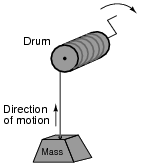A physicist would likely look at this scenario as an example of energy exchange: the person turning the drum is expending energy, which in turn is being stored in the mass in potential form.

Suppose now that the person stops turning the drum and instead engages a brake mechanism on the drum so that it reverses rotation and slowly allows the mass to return to ground level. Once again, a physicist would view this scenario as an exchange of energy: the mass is now releasing energy, while the brake mechanism is converting that released energy into heat: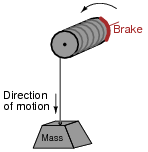In each of the above scenarios, draw arrows depicting directions of two forces: the force that the mass exerts on the drum, and the force that the drum exerts on the mass. Compare these force directions with the direction of motion in each scenario, and explain how these directions relate to the mass and drum alternately acting as energy source and energy load.

• #### Question 8

Draw the direction of current in this circuit, and also identify the polarity of the voltage across the battery and across the resistor. Then, compare the battery’s polarity with the direction of current through it, and the resistor’s polarity with the direction of current through it.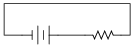What do you notice about the relationship between voltage polarity and current direction for these two different types of components? Identify the fundamental distinction between these two components that causes them to behave differently.

• #### Question 9

When an electric current travels through an electrical resistance, not only will there be a voltage “drop” across that resistance (V = IR), but there will also be energy dissipated by that resistance. We describe the rate of energy dissipation over time as power (P), and we express power in the unit of the watt (W).

Write the equation relating power to voltage and current, for an electrical resistance. Also, describe what physical form this dissipated energy usually takes.

• #### Question 10

What would happen if a wire having no resistance at all (0 Ω) were connected directly across the terminals of a 6-volt battery? How much power would be dissipated, according to Joule’s Law?Suppose I short-circuited a 6-volt battery in the manner just described and found that the wire used to make the short-circuit gets warm after just a few seconds of carrying this current. Does this data agree or disagree with your predictions?

• #### Question 11

How much electrical power is being dissipated by the light bulb in this circuit?In this circuit, identify which component is the source and which is the load.

• #### Question 12

Calculate the amount of power dissipated by this electric heating element, if the generator’s output voltage is 110 volts and the heater’s resistance is 2.5 ohms: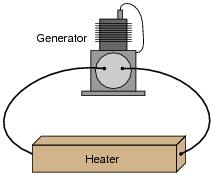Now, calculate the power dissipated by the same heater if the generator’s output voltage is doubled.

• #### Question 13

A DC electric motor transforms 1.75 kW of electrical power into mechanical form. If the motor’s operating voltage is 300 volts, how much current does it “draw” when operating at full load (full power output)?

Also calculate the motor’s output power in units of horsepower, assuming 100% efficiency.•Kirito Azuna April 07, 2022